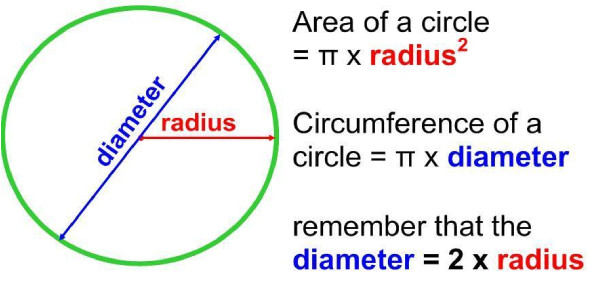# Circumference And Area Of Circle Quiz! Trivia

15 Questions | Attempts: 4766
ShareSettingsCan you pass this Circumference and Area of Circle Quiz? Some students get confused identifying which formulas are used when it comes to getting the circumference or area of a circle. The confusion comes in knowing which one to use the radius squared or not. In this quiz, you will get to see if you are among this league of students. Do give it a shot and see how well you do!

• 1.
Round to the nearest tenth. Find the circumference of a circle with a radius of 5.6 meters.
• A.

98.5

• B.

35.2

• C.

36.8

• D.

18.5

• 2.
Round to the nearest tenth. Find the circumference of a circle with a radius of 51.25 inches.
• 3.
Round to the nearest tenth. Find the circumference of a circle with a radius of 30.9 centimeters.
• A.

194.1

• B.

2998.1

• C.

97.0

• D.

61.8

• 4.
Round to the nearest tenth. Find the area of a circle with a diameter of 19 mm.
• 5.
Round to the nearest tenth. Find the area of a circle with a diameter of 25 yd.
• A.

157.0

• B.

256.3

• C.

1962.5

• D.

503.1

• 6.
Round to the nearest tenth. Find the area of a circle with a radius of 13.8 m.
• 7.
Round to the nearest tenth. Find the area of a circle with a diameter of 46.2 cm.
• A.

6702.1

• B.

1587.3

• C.

1675.5

• D.

145.1

• 8.
Round to the nearest tenth. Find the area of a circle with a radius of 3.25 in.
• 9.
Round to the nearest tenth. Find the area of a circle with a diameter of 6.8 meters.
• A.

36.3

• B.

36.4

• C.

145.2

• D.

145.1

• 10.
Round to the nearest tenth. A sprinkler can spray water 10 feet in all directions. How much area can sprinkler water?
• A.

62.8 sq.feet

• B.

36.3 sq.feet

• C.

314.0 sq.feet

• D.

98.9 sq.feet

• 11.
Round to the nearest tenth. What is the number of square meters of a half circle whose diameter is 8 m?
• A.

50.2

• B.

45.6

• C.

15.3

• D.

25.1

• 12.
Round to the nearest tenth. Olivia used a stick to draw a circle in the sand. The circle was 4 meters in radius. How many square meters were inside the circle?
• A.

50.2

• B.

25.1

• C.

45.6

• D.

13.6

• 13.
• A.

307.7

• B.

615.4

• C.

87.9

• D.

14.0

• 14.
Round to the nearest tenth. Ryan used a drafting compass to draw a circle on the paper. The circle was 12 cm in radius. How many square centimeters were inside the circle?
• A.

144.2

• B.

425.1

• C.

388.7

• D.

452.2

• 15.
Round to the nearest tenth. A pizza was cut into 8 equal pieces. John's crust on his piece measured 5 inches. From the point of his slice to the edge of his crust, how many inches long was John's slice?
• A.

78.5

• B.

40.0

• C.

12.7

• D.

6.4

## Related TopicsBack to top
×

Wait!
Here's an interesting quiz for you.